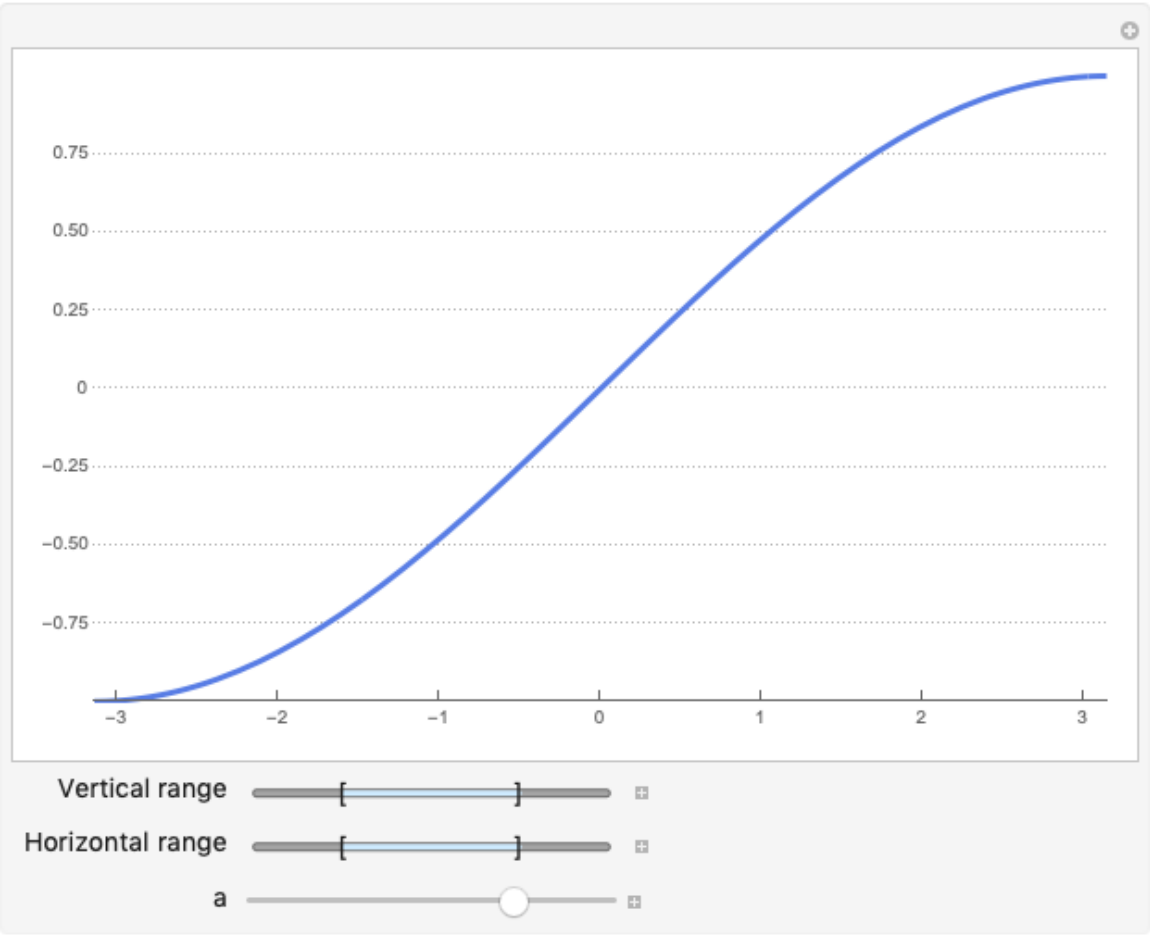#Function Repository Resource:

# ManipulatePlot

Generate a plot in which the plot ranges and function parameters can be manipulated dynamically

Contributed by: Wolfram|Alpha Math Team
 ResourceFunction["ManipulatePlot"][expr,{x,lo,hi},{a,min,max},…] returns a manipulable plot of expr on the domain lo≤x≤hi with symbolic parameters varying on the given domains. ResourceFunction["ManipulatePlot"][{expr1,expr2,…},…] returns a manipulable plot of all of the expri.

## Details and Options

ResourceFunction["ManipulatePlot"] has syntax that merges those of Manipulate and Plot where the independent variable for plotting x, along with its bounds, forms the second argument. Subsequent parameters become Manipulate variables.
ResourceFunction["ManipulatePlot"] takes the options of both Manipulate and Plot.
Both the horizontal and vertical plot ranges are adjustable in the generated plot.

## Examples

### Basic Examples

Plot a basic function allowing manipulation of the parameters and plot range:

 In:=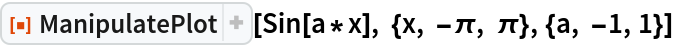Out=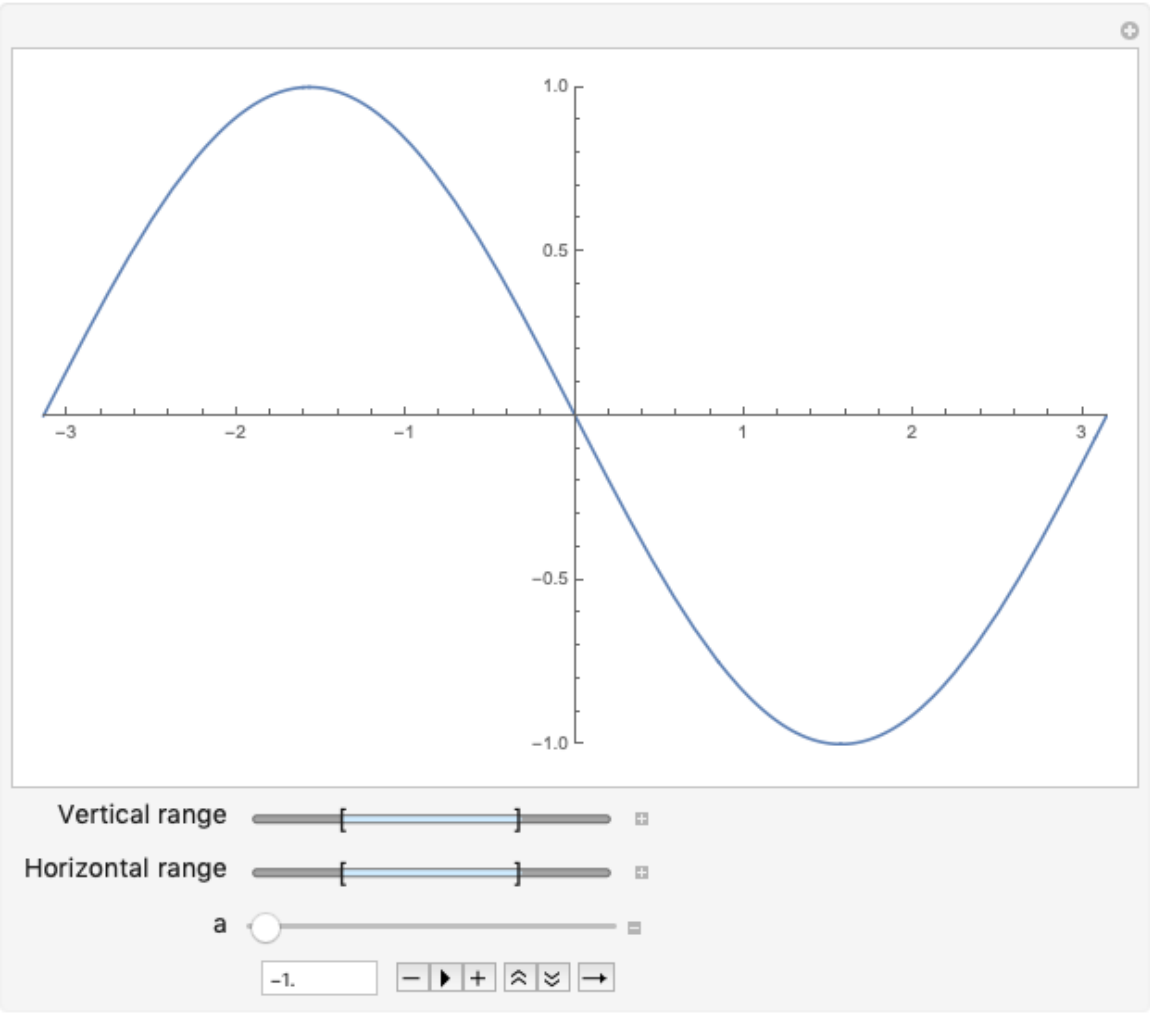### Scope

Initialize a parameter using Manipulate syntax:

 In:=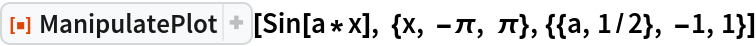Out=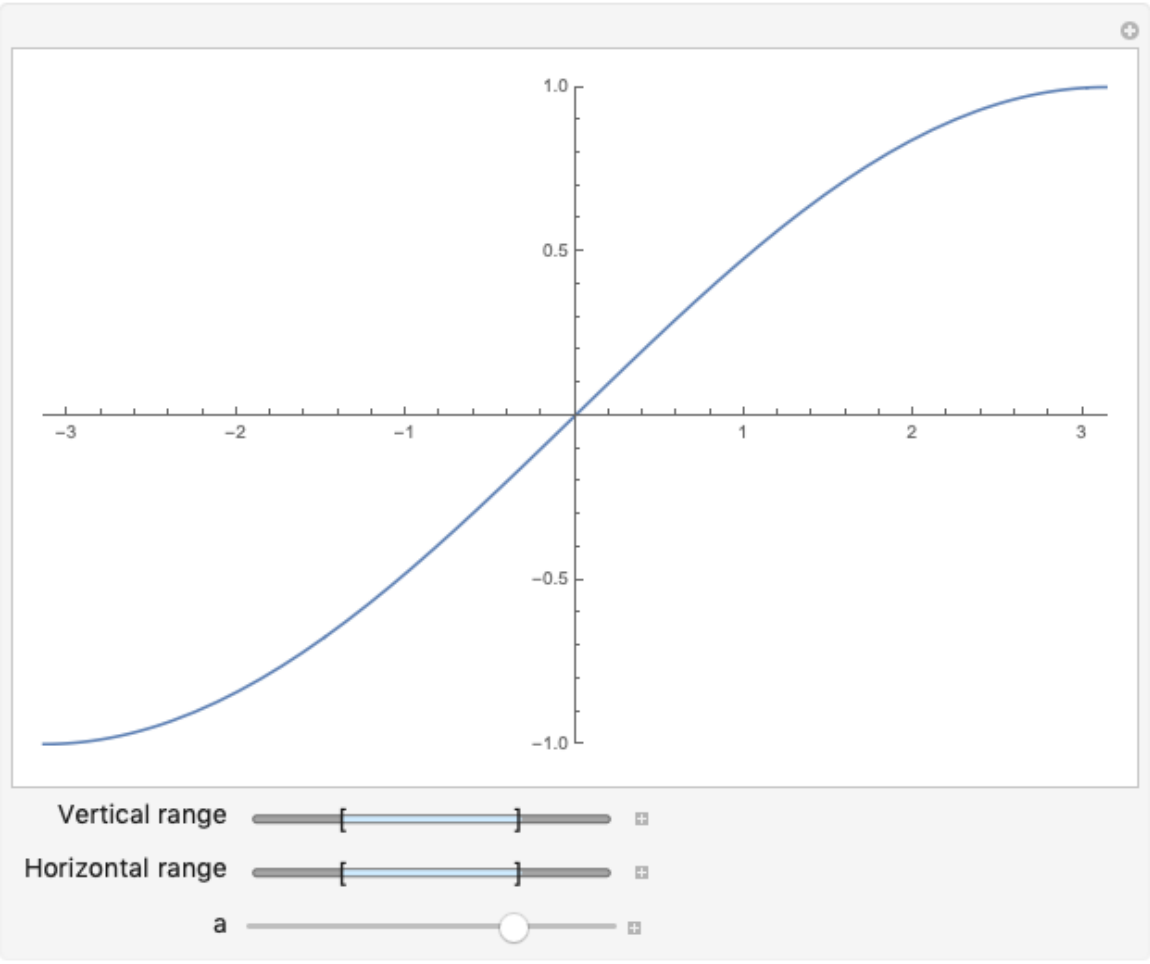ManipulatePlot can support multiple curves and take many parameters:

 In:=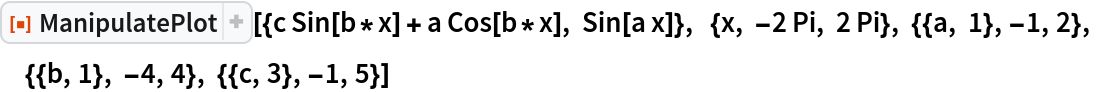Out=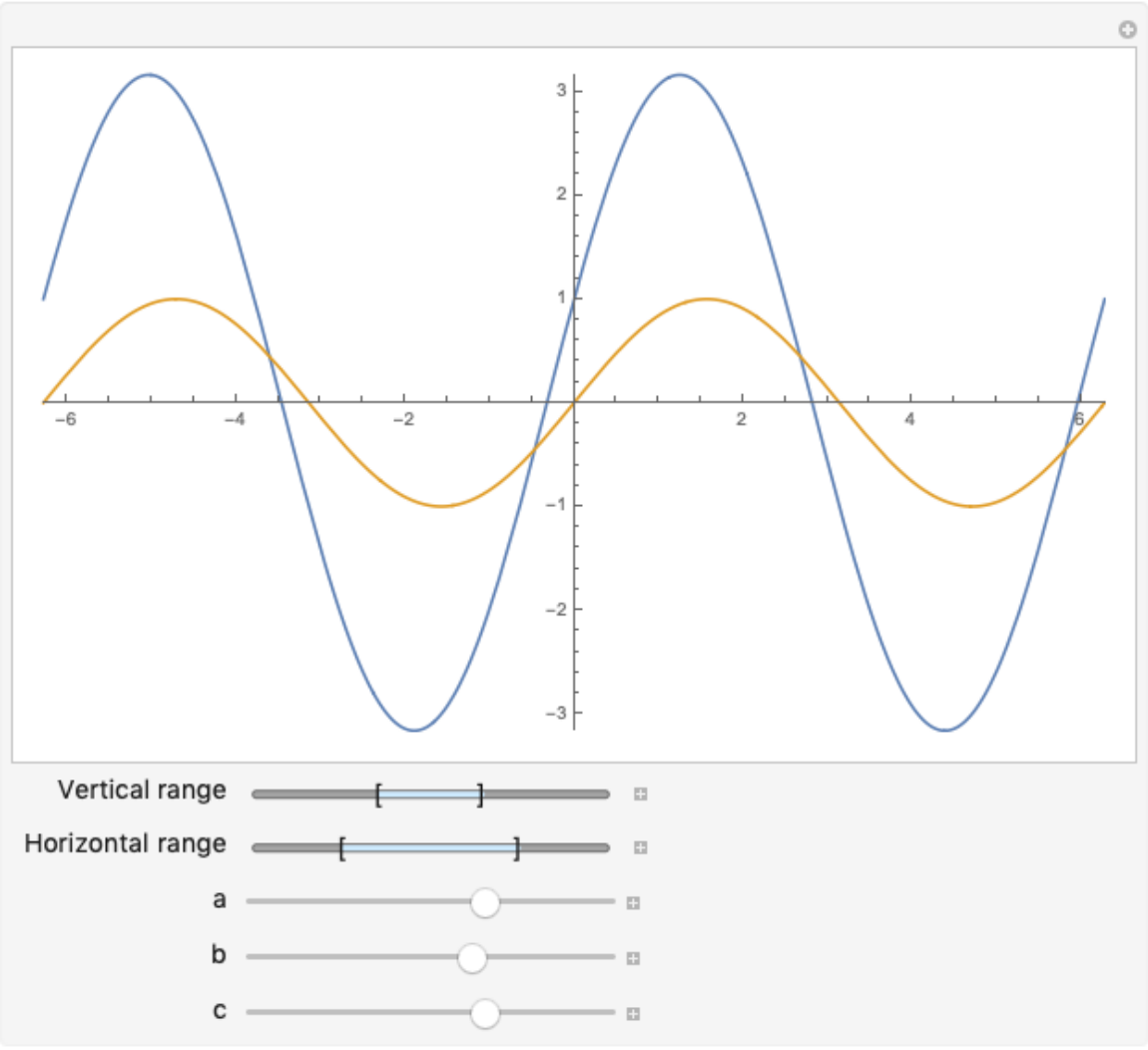### Options

ManipulatePlot accepts options for both Plot and Manipulate:

 In:=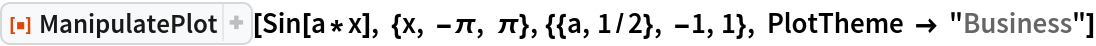Out=